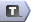# Logic in AMPL

## Relational Operators

Relational operators are used to compare two expressions. They are most commonly used in constraints, but not exclusively. The relational operators are:

Expression Meaning
< Less than
<= Less than or equal to
> Greater than
>= Greater than or equal to
== Equal to
<> Not equal to

## Logical Operators

Logical operators are used to combine logical expressions. They are most commonly used in conditional statements, conditional structures and conditional loops. The logical operators are `not`, `and` and `or`. They are used as shown in the table below:

Expression Result
`not ` True if is false, false if is true
` and ` True if and are both true, otherwise false
` or ` True if either or are true, otherwise false

## Logical Expressions

Logical expressions are expressions that will evaluate to either true or false. Logical expressions are usually defined in terms of the relational operators:

`Lower[r] <= sum {i in INGREDIENTS} Contributes[r, i] * Amount[i] `

`sum {s in SURFBOARDS} Recipe[m, s] * Production[s] <= Supply[m]; `

However, there are some special logical expressions in AMPL for use with sets:

1. in is true if is a member of ;
2. not in is false if is a member of ;
3. exists { in } is true if some in has being true;
4. forall { in } is true if all in have being true;
5. within is true if all the elements in are in ;
6. not within is true if some element in is not in .

Logical expressions can be built up from other logical expressions, binary parameters and logical operators.

## Conditional Expressions

A conditional expression is very much like the `IF` function in Microsoft Excel:

`param ifvalue := if  then                                     [else                    ]; `

## Conditional Structures

A conditional structure is the same as the classical if_-_then_-_else statement in programming languages like MATLAB, Fortran, Visual Basic and C++:

`if  then   ; [else   ;] `
`if  then {    } [else {    }] `

## Binary Parameters

In AMPL we can create binary parameters by using the `binary` keyword in the parameter declaration:

`param stillSearching binary; `

Binary parameters are used in a similar way to boolean variables (in Matlab, C, etc) and logical variables (Fortran). If a binary parameter has the value 0 this is equivalent to false, and 1 is equivalent to true. Binary parameters can be used with conditional expressions to hold a true/false result from a logical expression:

`param isGreater binary;  let isGreater := if 4 > 5 then 1 else 0; # isGreater = 0 (false) let isGreater := if 6 > 5 then 1; # else 0 is the default, isGreater = 1 (true) `
so the syntax is
`let  := if  then 1; `

You can also set binary parameters within conditional structures

`binary ;  if  then   let  := 1; else   let  := 0; `

Binary parameters may be used in logical expressions or as the condition in a conditional statement or conditional structure. They are very useful for building complex conditional statements or structures:

`Some example from depth first searching or column generation `
Coming soon! and controlling conditional loops:
`Some example from depth first searching or column generation `
Coming soon!

-- TWikiAdminGroup - 18 Mar 2008

Topic revision: r7 - 2009-09-18 - MichaelOSullivanCopyright © 2008-2023 by the contributing authors. All material on this collaboration platform is the property of the contributing authors.
Ideas, requests, problems regarding TWiki? Send feedback